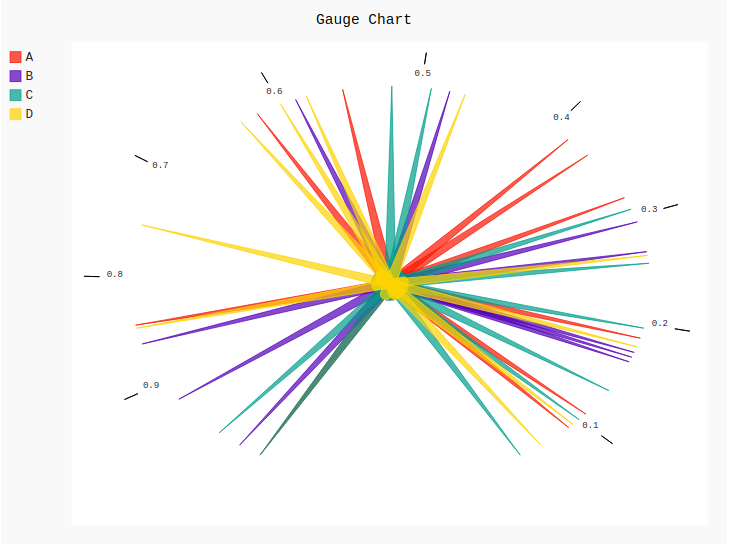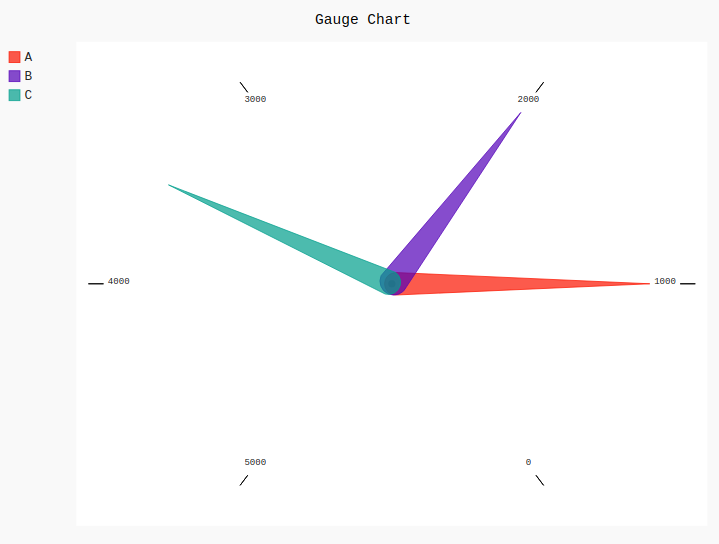# Gauge Chart in pygal

Pygal is a Python module that is mainly used to build SVG (Scalar Vector Graphics) graphs and charts. SVG is a vector-based graphics in the XML format that can be edited in any editor. Pygal can create graphs with minimal lines of code that can be easy to understand and write.

## Gauge Charts

Gauge charts are also known as dial charts or speedometer charts. This type of chart is often used in executive dashboard reports to show key business indicators. Gauge charts are much useful for comparing the values between a small number of variables either by using multiple needles on the same gauge or by using multiple gauges. It shows the maximum, the minimum, and the present value of data being analyzed. It can be created using the `Gauge()` method.

Syntax:

`gauge_chart = pygal.Gauge()`

Example 1:

 `# importing pygal ` `import` `pygal ` `import` `numpy ` ` `  ` `  `# creating the chart object ` `gauge ``=` `pygal.Gauge() ` ` `  `# naming the title ` `gauge.title ``=` `'Gauge Chart'`         ` `  `# Random data ` `gauge.add(``'A'``, numpy.random.rand(``10``)) ` `gauge.add(``'B'``, numpy.random.rand(``10``)) ` `gauge.add(``'C'``, numpy.random.rand(``10``)) ` `gauge.add(``'D'``, numpy.random.rand(``10``)) ` ` `  `gauge `

Output:Example 2:

 `# importing pygal ` `import` `pygal ` ` `  `# creating the chart object ` `gauge ``=` `pygal.Gauge() ` ` `  `# naming the title ` `gauge.title ``=` `'Gauge Chart'`      ` `  `gauge.``range` `=` `[``0``, ``5000``] ` ` `  `# Random data ` `gauge.add(``'A'``, ``1000``) ` `gauge.add(``'B'``, ``2000``) ` `gauge.add(``'C'``, ``3500``) ` ` `  `gauge `

Output:My Personal Notes arrow_drop_upIf you like GeeksforGeeks and would like to contribute, you can also write an article using contribute.geeksforgeeks.org or mail your article to contribute@geeksforgeeks.org. See your article appearing on the GeeksforGeeks main page and help other Geeks.

Please Improve this article if you find anything incorrect by clicking on the "Improve Article" button below.

Article Tags :

Be the First to upvote.

Please write to us at contribute@geeksforgeeks.org to report any issue with the above content.